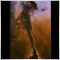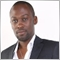# Next Timeframe271

I need a function to calculate the next timeframe up from the current chart. So if I'm trading on a 30 tf I'd want it to return 60. Thanks.201

Let's have a look at :

 int Period( )

Returns the amount of minutes determining the used period (chart timeframe).

 PERIOD_M1 1 1 minute. PERIOD_M5 5 5 minutes. PERIOD_M15 15 15 minutes. PERIOD_M30 30 30 minutes. PERIOD_H1 60 1 hour. PERIOD_H4 240 4 hour. PERIOD_D1 1440 Daily. PERIOD_W1 10080 Weekly. PERIOD_MN1 43200 Monthly. 0 (zero) 0 Timeframe used on the chart.271

And how would I use this valuable information to formulate a function?271

This is what I've got so far:

int Test()
{
int TimeFrames = { 1,5,15,30,60,240,1440,10080,43200 };
for(int i=0; i<=9; i++)
if(TimeFrames[i]==Period()) return(TimeFrames[i+1]);
}271

And this is correct....

I appreciate the info but please read the question before replying. Knowledge of Period() does not preclude a knowledge of arrays and conditional statements. I asked hoping that their was a built-in array for timeframes.79

hey,

I modified your code a little:

int Test()
{
int TimeFrames = { 1,5,15,30,60,240,1440,10080,43200,43200 };
for(int i=0; i<9; i++)

if( TimeFrames[i] == Period() ) return(TimeFrames[i+1]);
}

The above should do what you asked for. I dont know what you would like to return above the monthly timeframe, I put the same number (monthly) as the next one - you may change it according to your needs...

thank you

Automated (automatedfx@gmail.com)

--
http://www.forexmosaic.com/ - free publishing, analyzing and portfolio building service271

Thanks Automated. I didn't even think about the monthly. I just changed the next number to 0 since there is nothing higher than monthly; same premise.10

```//+---------------------------------------------------------------------+
//| subGetNextTimeFrame function - returns the next timeframe           |
//+---------------------------------------------------------------------+
int subGetNextTimeFrame()
{
int TimeFrames = { 1,5,15,30,60,240,1440,10080,43200 };
int CurrentTimeIndex = ArrayBsearch(TimeFrames,_Period,8,0,MODE_ASCEND);
return((ENUM_TIMEFRAMES) TimeFrames[CurrentTimeIndex+1]);
}```